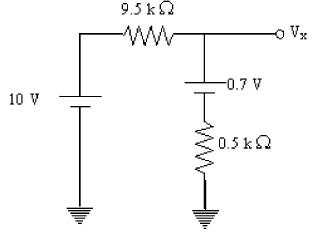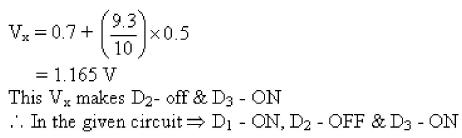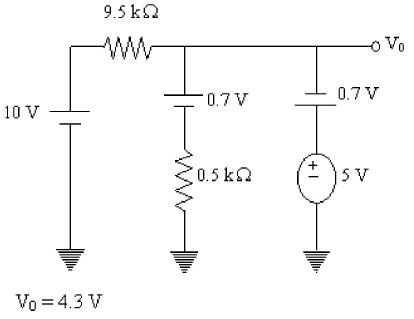# Electrical Engineering (EE) : Mock Test 1 For GATE

## 65 Questions MCQ Test Mock Test Series for Electrical Engineering (EE) GATE | Electrical Engineering (EE) : Mock Test 1 For GATE

Description
This mock test of Electrical Engineering (EE) : Mock Test 1 For GATE for GATE helps you for every GATE entrance exam. This contains 65 Multiple Choice Questions for GATE Electrical Engineering (EE) : Mock Test 1 For GATE (mcq) to study with solutions a complete question bank. The solved questions answers in this Electrical Engineering (EE) : Mock Test 1 For GATE quiz give you a good mix of easy questions and tough questions. GATE students definitely take this Electrical Engineering (EE) : Mock Test 1 For GATE exercise for a better result in the exam. You can find other Electrical Engineering (EE) : Mock Test 1 For GATE extra questions, long questions & short questions for GATE on EduRev as well by searching above.
QUESTION: 1

### Statement: All bags are cakes. All lamps are cakes. Conclusions: I. Some lamps are bags. II. No lamp is bag. Deduce which of the above conclusion logically follows statements:

Solution:

Conclusion I and II are complementary. So either conclusion I or conclusion II follows the statement.

QUESTION: 2

### Man does not live by __________ alone.

Solution:

Man does not live by bread alone

QUESTION: 3

### Extreme focus on syllabus and studying for tests has become such a dominant concern of Indian students that they close their minds to anything __________ to the requirements of the exam.

Solution:

Extraneous – irrelevant or unrelated to the subject being dealt with

QUESTION: 4

Select the pair that best expresses a relationship similar to that expressed in the pair
LIGHT : BLIND

Solution:
QUESTION: 5

If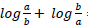= log(a + b), then:

Solution:

RHS : Log a + Log b = Log ab

For LHS : Log a/b + Log b/a

= Log ((a/b) *(b/a))

=Log (ab/ab) =1

LHS = RHS

∴1=a+b

QUESTION: 6

In an exam, the average was found to be 50 marks. After deducting computational errors the marks of the 100 candidates had to be changed from 90 to 60 each and average came down to 45 marks. Total number of candidates who took the exam was:

Solution:

Let number of candidates be n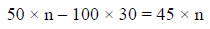n = 600

QUESTION: 7

A solid 4cm cube of wood is coated with red paint on all the six sides. Then the cube is cut into smaller 1cm cubes. How many of these 1cm cubes have no colour on any side?

Solution:

Number of cubes with 3 faces red = 8
Number of cubes with 2 faces red = 24
Number of cubes with 1 face red = 24
Number of cubes with no face red = 64-(24+24+8) = 8

QUESTION: 8

One of the warmest winters on record has put consumers in the mood to spend money. Spending is to be the strongest in thirteen years. During the month of February, sales of existing single family homes hit an annual record rate of 4.75 million. This paragraph best supports the statement that:

Solution:
QUESTION: 9

The age of father is 4 times more than the age of his son Amit. After 8 years, he would be 3 times older than Amit. After further 8 years, how many times will he be older than Amit?

Solution:

Let Amit’s age be n years
Age of his father = (4*n) = 4n years
After 8 years:
Amit’s age = n + 8
Father’s age =
3(n + 8) = 4n + 8
3n + 24 = 4n + 8
16 = n
n = 16 years
After further 8 years:
Amit’s age = 24 years
Father’s age = 4n +16 = 80 years
So father is 2.5 times older than Amit.

QUESTION: 10

Pipe A, B and C are kept open and together fill a tank in t minutes. Pipe A is kept open throughout, pipe B is kept open for the first 10 minutes and then closed. Two minutes after pipe B is closed, pipe C is opened and is kept open till the tank is full. Each pipe fills an equal share of the tank. Furthermore, it is known that if pipe A and B are kept open continuously, the tank would be filled completely in t minutes. Find t?

Solution:

A is kept open for all t minutes and fills one-third the tank. Or, A should be able to fill the entire tank in '3t' minutes.

A and B together can fill the tank completely in t minutes. A alone can fill it in 3t minutes.

A and B together can fill 1/t  of the tank in a minute. A alone can fill 1/3t of the tank in a minute. So, in a minute, B can fill 1/t - 1/3t=2/3t. Or, B takes 3t/2 minutes to fill an entire tank.

To fill one-third the tank, B will take t/2 minutes. B is kept open for t -10 minutes.

t/2 =  t - 10,

∴ t = 20 minutes.

A takes 60 minutes to fill the entire tank, B takes 30 minutes to fill the entire tank. A is kept open for all 20 minutes. B is kept open for 10 minutes.
C, which is kept open for 8 minutes also fills one-third the tank. Or, C alone can fill the tank in 24 minutes.

*Answer can only contain numeric values
QUESTION: 11

The value of ‘V’ from the given below circuits is_____________ volts.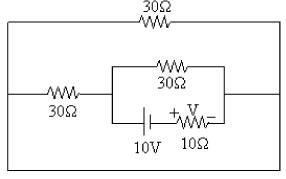Solution:

By redrawing the given circuit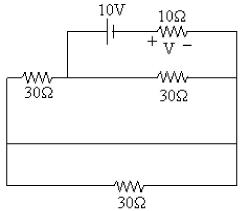Current flowing through 30Ω is zero. So circuits becomes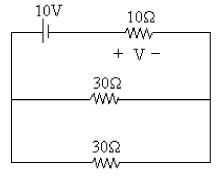Current flowing through 10Ω resistor is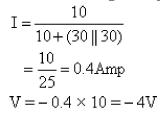QUESTION: 12

For eliminating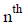Harmonics from the emf generated the coil span must be.

Solution: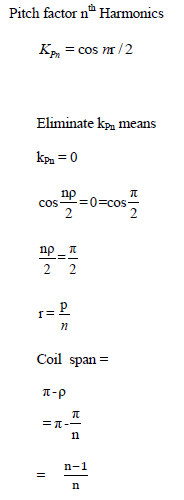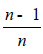, Full pitch

QUESTION: 13

Determine the value of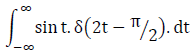Solution:

Let x=2t
t=x/2
dt=dx/2
∴ Integral becomes

= sin(x/2) * δ(x-(pie/2)) * (dx/2)
∴ (1/2) *sin(pie/4) =1/(2√2)

QUESTION: 14

In HVDC converter stations equipment using thyristor it is necessary to use a large number of thyristors in series because

Solution:
QUESTION: 15

The holding current of a SCR is 18mA. Its latching current will be

Solution:

Ratio between latching current to  holding current is 2-3 times.

QUESTION: 16

In a 132 kV system, the phase to ground capacitance is 0.01 µF and the inductance is 4H. Calculate the voltage appearing across the electrodes of C.B. If a magnetizing current at 5 amp is interrupted. Calculate the critical resistance to be connected, to eliminate restriking.

Solution: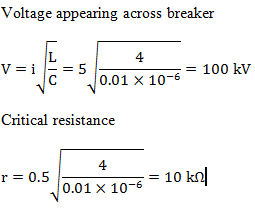QUESTION: 17

A phase lead compensating network has its transfer function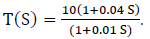The maximum phase lead occurs at a frequency of:

Solution:

Comparing with the standard phase lead compensating network,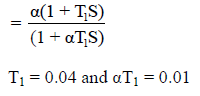So, maximum phase lead occurs at frequency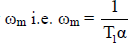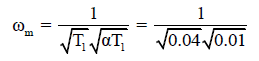QUESTION: 18

The time taken for the output to settle within 2% of step input for the control system represented by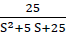is given by

Solution:

Comparing the above transfer function with the standard second order transfer function.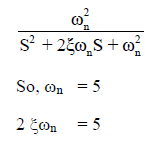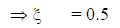Settling time for 2% tolerance band =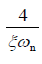=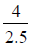= 1.6 sec

QUESTION: 19

When 30V dc is applied to the vertical deflection plates of CRO, the bright spot moves 1cm away from the center if 30V (rms) is applied then the picture on screen

Solution:
QUESTION: 20

A compound dc generator is delivering full load current at a terminal voltage of 230V. It’s
series field winding get’s short circuit. If it’s terminal voltage becomes.
1. More than 230V it is over compounded
2. More than 230V it is differential compound
3. Less than 230V it is over compounded
4. Less than 230V it is differential compounded
5. Less than 230V it is level compounded
The correct statement’s are?

Solution:
QUESTION: 21

An (star connected) Alternator is connected to Delta-star transformer with start neutral earthed. On the star side of transformer a single line to ground fault occurs. This is

Solution:

If the winding is delta connected, it does not associated with 0 sequence component current, but only +ve, -ve sequence components exist. Also only +ve & -ve sequence components exist only in line to line fault

QUESTION: 22

Snubber circuit is used to limit the rate of

Solution:
QUESTION: 23

The unit of mobility is:

Solution:

Mobility= drift velocity/Electric field

QUESTION: 24

In the circuit shown in figure, a Silicon transistor with VBE = 0.7 V and βdc = 100 is used. The Q-point is established at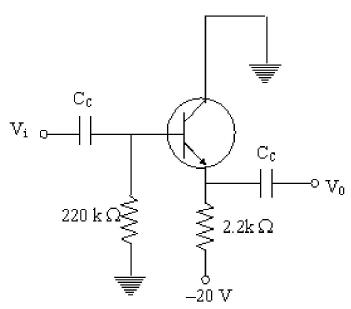Solution:

DC equivalent of the given circuit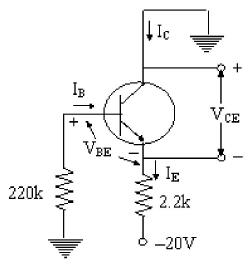Step (1): KVL for B-E loop
0 - IB 220 k - VBE - IE (2. 2k) + 20 V =0
=> IB (220k) + (1 + β) I2.2k = 19.3 V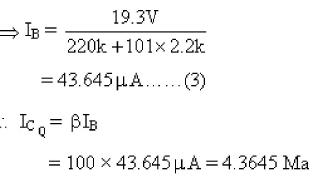Step (2): KVL for C-E loop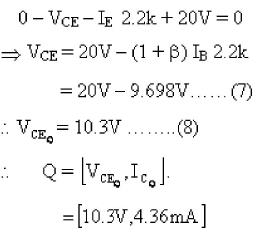QUESTION: 25

A power supply has a full load voltage of 24V. What is its no-load voltage for 5% regulation.

Solution:

Voltage - Regulation,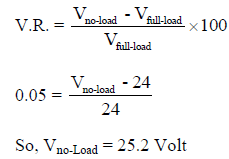QUESTION: 26

Assume that the op-amp as the circuits shown below is ideal. Find the current passing through the 2kΩ resistor in the circuit.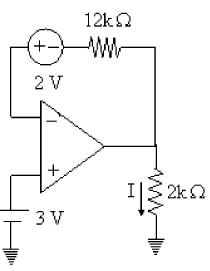Solution: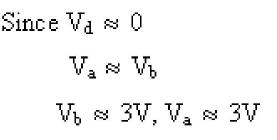But the current entering into the op-amp terminals is zero. So current through 12k is zero.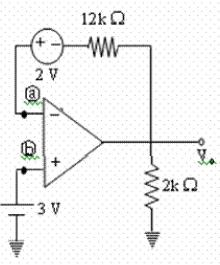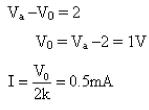QUESTION: 27

The diode in the circuit given below has VON = 0.7 V but is ideal otherwise. The current (in mA) in the 4kΩ resistor is______ mA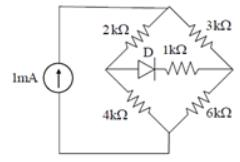Solution:

Correct answer is 0.6 Given circuit is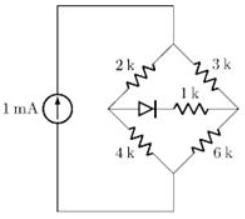Here, we have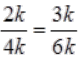So bridge is balanced, and hence, no current will flow through diode. The equivalent circuit is shown below.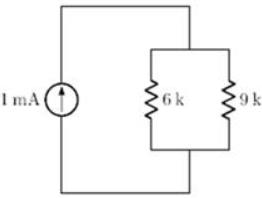Current through 4kΩ resistor is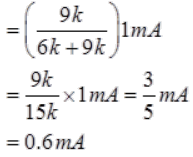QUESTION: 28

The system of linear equations:
3x + 4y = 6
2x + 3y = 5
has

Solution:

Auguemented matrix,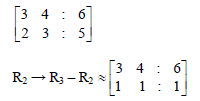Since rank of matrix A as well as auguemented matrix is 2, therefore it has unique solution

QUESTION: 29
Which of the following is a solution to the differential equation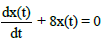Solution: (D + 8) x(t) = 0
D = -8,
So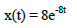QUESTION: 30
The value of the integral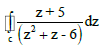where C is the circle |z| = 1
Solution: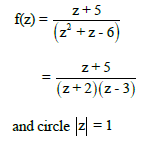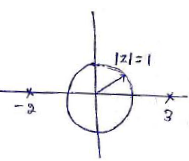Since both the poles lie outside the unit circle therefore according to Cauchey – Integral, the integration is zero
QUESTION: 31

A fair dice is tossed two times. The probability that the second results in a value that is higher than the first toss is:

Solution:

In the first toss, results can be 1, 2, 3, 4, 5
For 1, the second toss results can be 2, 3, 4, 5, 6
For 2, the second toss results can be 3, 4, 5, 6
For 3, the second toss results can be 4, 5, 6
For 4, the second toss results can be 5, 6
For 5, the second toss results can be 6
So the required probability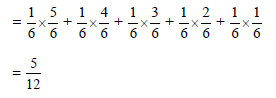QUESTION: 32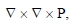where P is a vector is equal to :

Solution:

Gauss divergence theorem will explain the given expression.

QUESTION: 33

Identify the instruction that affects only Carry Flag.

Solution:
QUESTION: 34

The initial of MOD-16 down counter is 0110. After 37 clock pulses, the state of the counter will be:

Solution: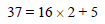After 37 clock pulses, the state of MOD-16 DOWN counter will be five states below the present state.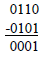QUESTION: 35

12 MHz clock frequency is applied to a cascaded counter of modulus-3 counter, modulus-4 counter, and modulus-5 counter. Determine the output frequency.

Solution: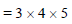= 60
So, frequency of the output signal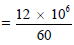= 200 KHz

QUESTION: 36

In the circuit shown, the power supplied by the voltage source is: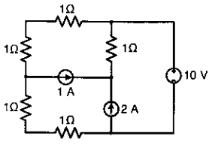Solution:

There is no current flowing through voltage source that's why the power delivered by voltage source is zero.

*Answer can only contain numeric values
QUESTION: 37

Total energy stored on network in kjoules at steady state is _______.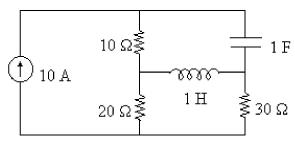Solution:

At steady state inductor behaves as short circuit and capacitor behaves as open circuits.
By drawing the circuit at steady state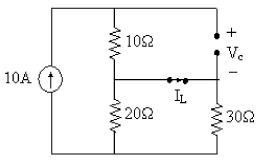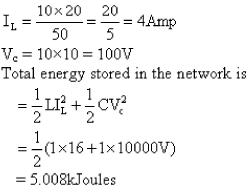QUESTION: 38

If the current changes from 3A to 5A in 2s and the emf is 10V. Calculate the inductance.

Solution:

We know that:
emf=L(i2-i1)/t
Substituting the values from the question we get L=10H.

QUESTION: 39

The output y(t) of a continuous – time system S for the input x(t) is given by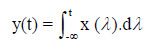Which one of the following is correct?

Solution:

For a continuous time system,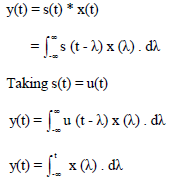Therefore s(t) = u(t)
Which is linear and time-invariant

QUESTION: 40
What is the output of the system with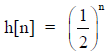u[n] in response to the input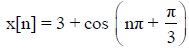Solution: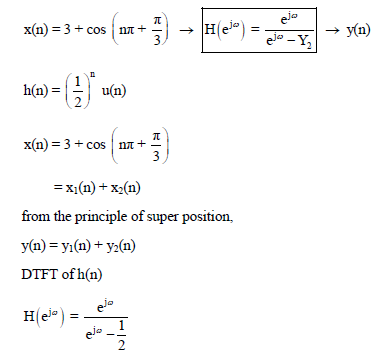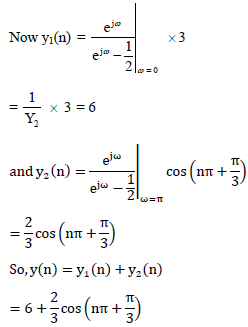QUESTION: 41
The 3-dB bandwidth of the low-pass filter having impulse response is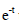u(t) is :
Solution: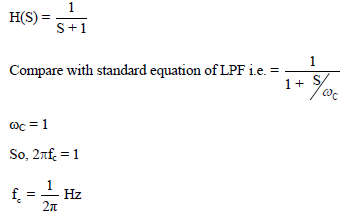QUESTION: 42
What is the inverse fourier transform of u(w) ?
Solution: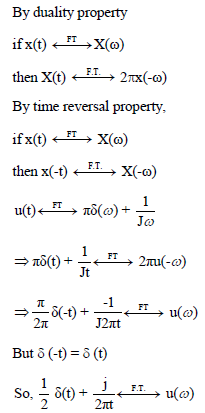QUESTION: 43

Consider the following block diagram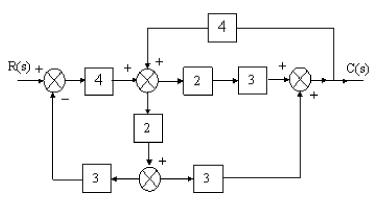Overall gain C(S)/R(s) of the above system is

Solution: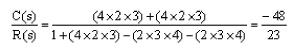QUESTION: 44

"What is the inverse lap lace transform ofSolution:
QUESTION: 45

Determine the value of K for a unity feedback system with G(s) =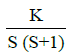to have a peak overshoot of 50%

Solution: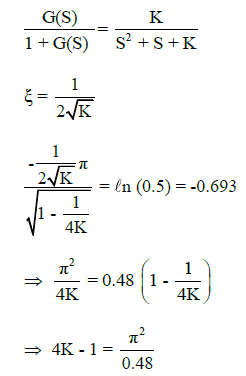4K = 21.56
= K = 5.39

QUESTION: 46

A there phase alternator is wound with a 60 degree phase-spread armature windings and develops 300 kVA. If the armature is reconnected utilizing all the coils for single phase operation with a phase spread of 180 degrees, the new rating of the machine is

Solution: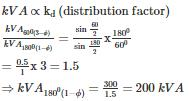QUESTION: 47

The flux density distribution for 50 Hz cylindrical rotor alternator is B(q) = sin q+ 0.3sin3q+ 0.2sin5q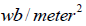where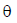is measured from neutral axis,alternator has pole pitch 40 cm and length 35 cm, stator coil span is four-fifth of the pole pitch. Find the emf generated in one turn coil?

Solution:

Peak flux density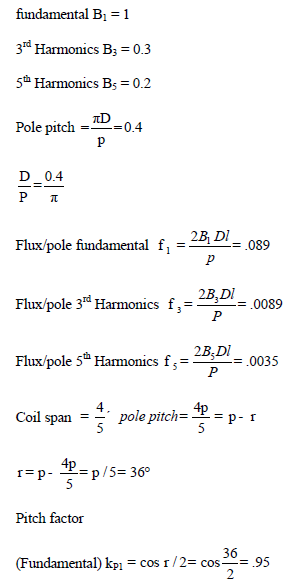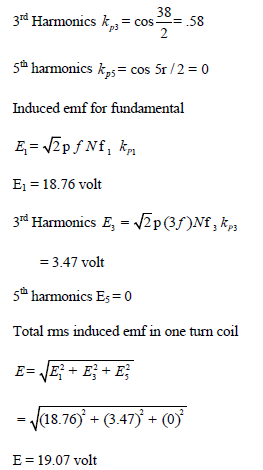QUESTION: 48

If the current changes from 5A to 3A in 2 seconds and the inductance is 10H, calculate the emf.

Solution:

We know that:
emf=L(i2-i1)/t
Substituting the values from the question, we get emf=10V.

QUESTION: 49

Find the type of feedback topology for the given circuit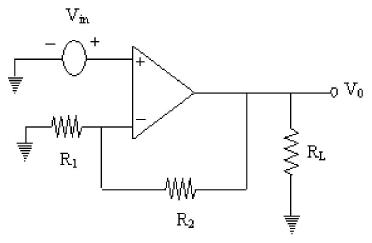Solution: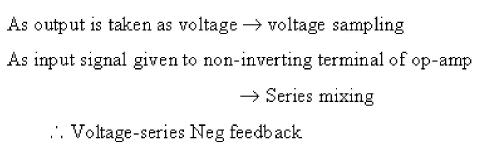QUESTION: 50

The power factor of pure resistive circuit is

Solution:

For the purely resistive circuit, the power factor is 1 (perfect), because the reactive power equals zero.

QUESTION: 51

A 400V / 100V, 10kVA two winding transformer is to be used as an autotransformer to supply a 400V circuit from 500V source when tested as two winding transformer at rated load .85 P.F. lag it’s efficiency is 97%. Determine efficiency as autotransformer at rated load and 0.85 power factor

Solution:

2 wdg TF : 10KVA, 400/100KV
Efficiency : 97%

Output Power = 10KVA × 0.85 = 8500W
Efficiency = Output / (Output + Losses) = 97%
Losses = 262.88W

Auto TF : Vh = 500V ; Ih=100A & VL= 400V ; IL=125A.   P=  (50KVA)

Output Power = 50KVA × 0.85 = 42500W
Losses (as same as 2Wdg) = 262.88W
Efficiency = Output / (Output + Losses) = 42500/( 42500 + 262.88)
= 99.38 %

*Answer can only contain numeric values
QUESTION: 52

A solid conductor of 4 cm radius is converted into four solid conductors of equal radius without changing net current rating. The four solid conductors are used to form a stranded conductor as follows.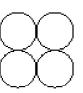GMR. of stranded conductor is____________ cm____________.

Solution: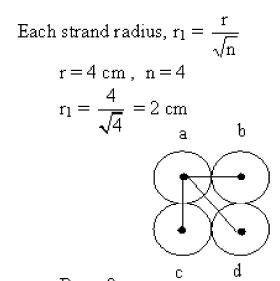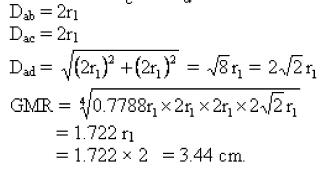QUESTION: 53

An amplifier using BJT has two identical stages each having a lower cut-off (3dB) frequency of 64Hz due to coupling capacitor. The emitter bypass capacitor also provides a lower cut-off (3dB) frequency due to emitter degeneration alone of 64Hz. The lower 3dB frequency of the overall amplifier is nearly

Solution:

So overall lower cut-off frequency,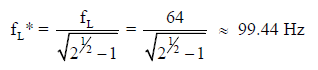= 100 Hz

QUESTION: 54

A 240V, 50 Hz supply feeds a highly inductive load of 50 ohm resistance through a half controlled thyristor bridge. When the firing angle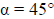the load power is:

Solution: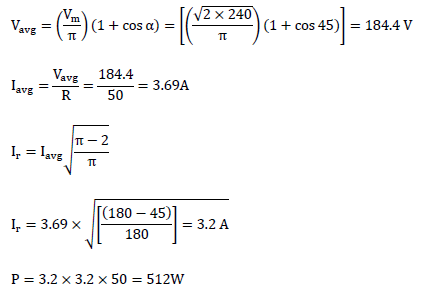QUESTION: 55

A pulse train with a frequency of 1MHz is counted using a mod-1024 ripple counter built with J–K flip-flops. For proper operation of the counter the maximum permissible propagation delay per flip-flop stage is:

Solution:

Here mod – 1024
So to count 1024 number of flip-flops requires
n = 10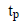of counter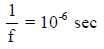So propagation delay per flip flop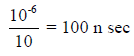QUESTION: 56

All flip-flops are triggered with negative edge.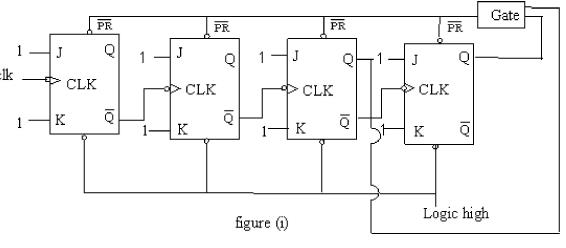The above counter shown in figure (1) is Mod 12 down counter. Then the Gate name is

Solution:

It starts with 1111 and after reaching 0011 the OR gate output becomes low. So, 'PR’ inputs get enabled.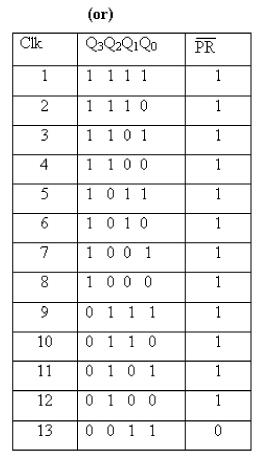If the input to the gate is 0011 then it preset's all the flip flops. The truth table for the gate is as follows.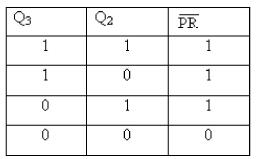From the above truth table we conclude that the given Gate is 'OR' Gate.

*Answer can only contain numeric values
QUESTION: 57

Minimum number of 2 input NOR gates needed to implement half sub tractor is ____________   ,

Solution:QUESTION: 58
A liner system has the transfer function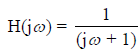When it is subjected to an input white noise process with a constant spectral density A, the spectral density of the output will be:
Solution: PSD of output =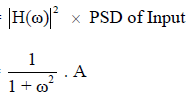QUESTION: 59

If a fault occurs near an impedance relay, the V/I ratio will be

Solution:

If the fault is away from the relay, then the impedance seen by the relay is more.

QUESTION: 60
A fault current of 2000A is passing on the primary side of 400/5 CT on the secondary side of the CT an inverse time over current relay is connected whose plug setting is at 50%. The PSM will be
Solution:
The pick up value of the relay is 5A, since the relay setting is 50%
Therefore the operating current of the relay is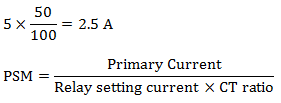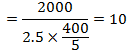QUESTION: 61

A d.c. to d.c. chopper operates from a 48 V battery source into a resistive load
of 24 Ω. The frequency of the chopper is set to 250Hz. When chopper on-time is 1 ms the load power is

Solution: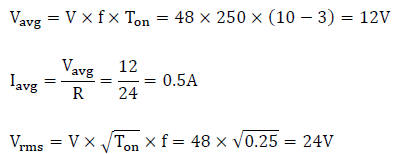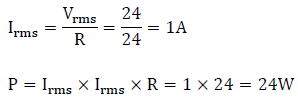QUESTION: 62

When converting 7,000 nA to microamperes, the result is

Solution:

1A = 1000(10-3)A.

1mA = 1000(10-6)A.

1uA = 1000(10-9)A.

1nA = 1000(10-12)A.

So, than the solution is: 7000nA = 7uA.

QUESTION: 63
The divergence of the vector field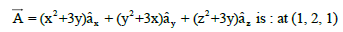Solution: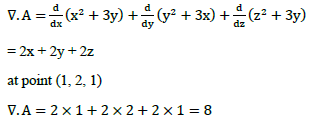QUESTION: 64
A solution for the differential equation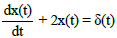with initial condition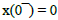is
Solution: Taking Laplace transform both sides we get,QUESTION: 65

The diodes in the circuit shown below have Vγ = 0.7 V. Then output voltage Vo is __________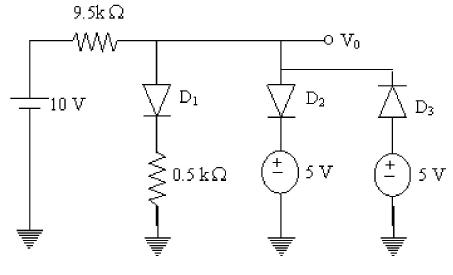Solution:

Apply open circuit test for the diodes and voltages across diodes are as
follows
D1 —> 10 V
D2 —> 5V
D3 -A -5V
So Dbecomes ON first